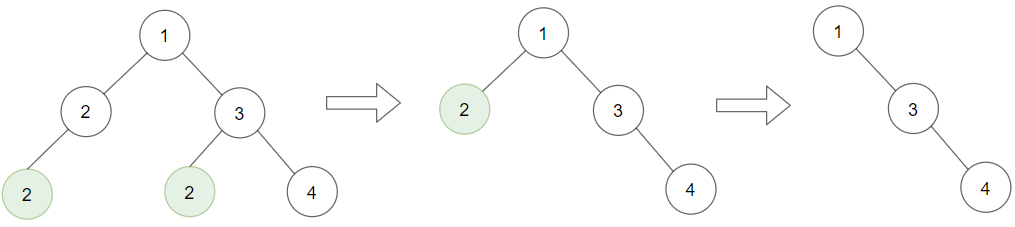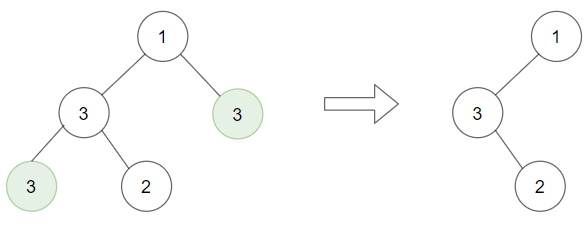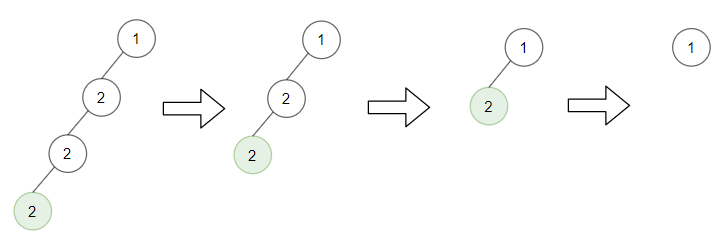Formatted question description: https://leetcode.ca/all/1325.html

# 1325. Delete Leaves With a Given Value (Medium)

Given a binary tree root and an integer target, delete all the leaf nodes with value target.

Note that once you delete a leaf node with value targetif it's parent node becomes a leaf node and has the value target, it should also be deleted (you need to continue doing that until you can't).

Example 1:Input: root = [1,2,3,2,null,2,4], target = 2
Output: [1,null,3,null,4]
Explanation: Leaf nodes in green with value (target = 2) are removed (Picture in left).
After removing, new nodes become leaf nodes with value (target = 2) (Picture in center).


Example 2:Input: root = [1,3,3,3,2], target = 3
Output: [1,3,null,null,2]


Example 3:Input: root = [1,2,null,2,null,2], target = 2
Output: 
Explanation: Leaf nodes in green with value (target = 2) are removed at each step.


Example 4:

Input: root = [1,1,1], target = 1
Output: []


Example 5:

Input: root = [1,2,3], target = 1
Output: [1,2,3]


Constraints:

• 1 <= target <= 1000
• Each tree has at most 3000 nodes.
• Each node's value is between [1, 1000].

Related Topics:
Tree

## Solution 1.

// OJ: https://leetcode.com/problems/delete-leaves-with-a-given-value/

// Time: O(N)
// Space: O(H)
class Solution {
private:
TreeNode* postorder(TreeNode *node, int target) {
if (!node) return NULL;
node->left = postorder(node->left, target);
node->right = postorder(node->right, target);
return !node->left && !node->right && node->val == target ? NULL : node;
}
public:
TreeNode* removeLeafNodes(TreeNode* root, int target) {
return postorder(root, target);
}
};


Java

/**
* Definition for a binary tree node.
* public class TreeNode {
*     int val;
*     TreeNode left;
*     TreeNode right;
*     TreeNode(int x) { val = x; }
* }
*/
class Solution {
public TreeNode removeLeafNodes(TreeNode root, int target) {
Map<TreeNode, TreeNode> childParentMap = new HashMap<TreeNode, TreeNode>();
queue.offer(root);
while (!queue.isEmpty()) {
TreeNode node = queue.poll();
TreeNode left = node.left, right = node.right;
if (left != null) {
childParentMap.put(left, node);
queue.offer(left);
}
if (right != null) {
childParentMap.put(right, node);
queue.offer(right);
}
}
boolean flag = true;
while (flag) {
flag = false;
Set<TreeNode> keySet = childParentMap.keySet();
Set<TreeNode> removeSet = new HashSet<TreeNode>();
for (TreeNode node : keySet) {
if (isLeaf(node) && node.val == target) {
TreeNode parent = childParentMap.get(node);
if (parent == null)
return null;
else {
if (node == parent.left)
parent.left = null;
else if (node == parent.right)
parent.right = null;
flag = true;
}
}
}
for (TreeNode node : removeSet)
childParentMap.remove(node);
}
if (isLeaf(root) && root.val == target)
root = null;
return root;
}

public boolean isLeaf(TreeNode node) {
return node != null && node.left == null && node.right == null;
}
}